# 监督学习：从高维观察预测输出变量

scikit-learn 中所有监督的估计量 都有一个用来拟合模型的 fit(X, y) 方法，和根据给定的没有标签观察值 X 返回预测的带标签的 ypredict(X) 方法。

## 最近邻和维度惩罚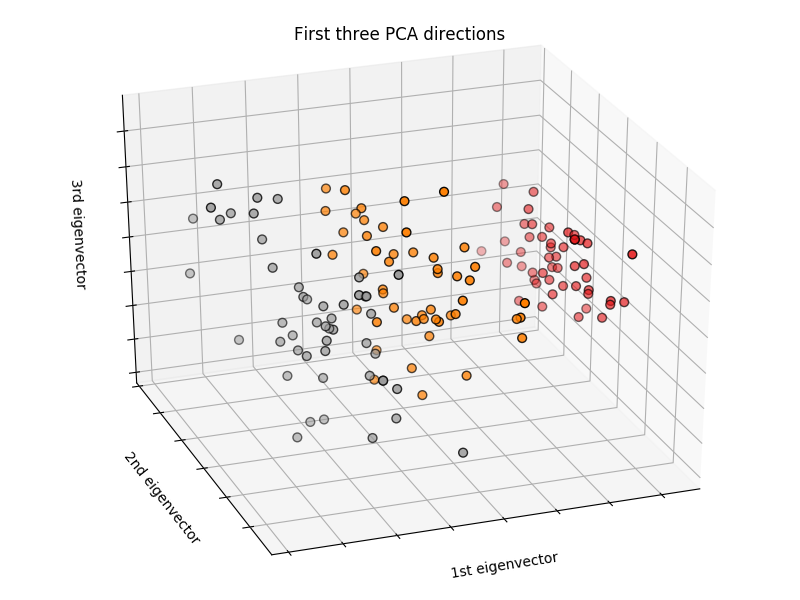>> import numpy as np
>> from sklearn import datasets
>> iris_X = iris.data
>> iris_y = iris.target
>> np.unique(iris_y)
array([0, 1, 2])


### K近邻分类器

KNN(k 最近邻)分类器示例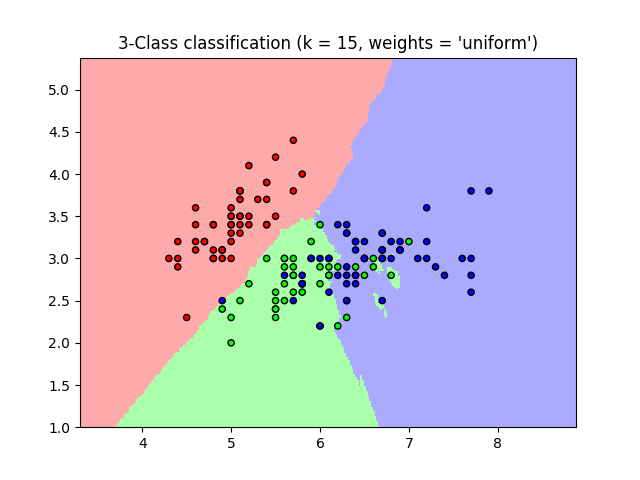>>> # 将鸢尾属植物数据集分解为训练集和测试集
>>> # 随机排列，用于使分解的数据随机分布
>>> np.random.seed(0)
>>> indices = np.random.permutation(len(iris_X))
>>> iris_X_train = iris_X[indices[:-10]]
>>> iris_y_train = iris_y[indices[:-10]]
>>> iris_X_test  = iris_X[indices[-10:]]
>>> iris_y_test  = iris_y[indices[-10:]]
>>> # 创建和拟合一个最近邻分类器
>>> from sklearn.neighbors import KNeighborsClassifier
>>> knn = KNeighborsClassifier()
>>> knn.fit(iris_X_train, iris_y_train)
KNeighborsClassifier(algorithm='auto', leaf_size=30, metric='minkowski',
metric_params=None, n_jobs=1, n_neighbors=5, p=2,
weights='uniform')
>>> knn.predict(iris_X_test)
array([1, 2, 1, 0, 0, 0, 2, 1, 2, 0])
>>> iris_y_test
array([1, 1, 1, 0, 0, 0, 2, 1, 2, 0])


## 线性模型：从回归到稀疏

>>> diabetes = datasets.load_diabetes()
>>> diabetes_X_train = diabetes.data[:-20]
>>> diabetes_X_test  = diabetes.data[-20:]
>>> diabetes_y_train = diabetes.target[:-20]
>>> diabetes_y_test  = diabetes.target[-20:]


### 线性回归

LinearRegression，最简单的拟合线性模型形式，是通过调整数据集的一系列参数令残差平方和尽可能小。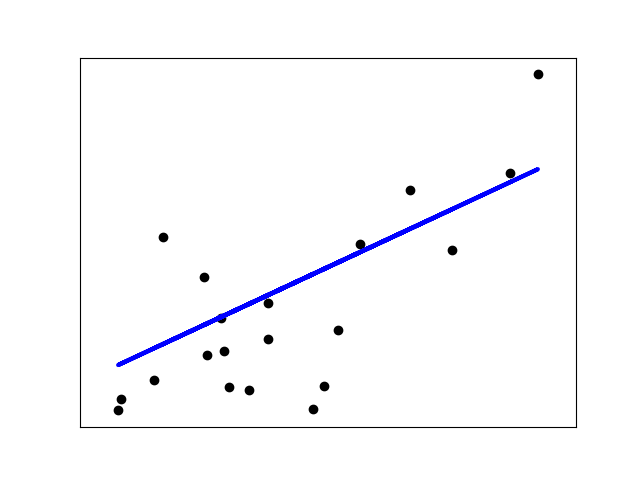Linear models: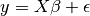•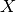：数据
•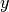：目标变量
•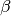：回归系数
•：观察噪声
>>> from sklearn import linear_model
>>> regr = linear_model.LinearRegression()
>>> regr.fit(diabetes_X_train, diabetes_y_train)
LinearRegression(copy_X=True, fit_intercept=True, n_jobs=1, normalize=False)
>>> print(regr.coef_)
[   0.30349955 -237.63931533  510.53060544  327.73698041 -814.13170937
492.81458798  102.84845219  184.60648906  743.51961675   76.09517222]

>>> # 均方误差
>>> np.mean((regr.predict(diabetes_X_test)-diabetes_y_test)**2)
2004.56760268...

>>> # 方差分数：1 是完美的预测
>>> # 0 意味着 X 和 y 之间没有线性关系。
>>> regr.score(diabetes_X_test, diabetes_y_test)
0.5850753022690...


### 收缩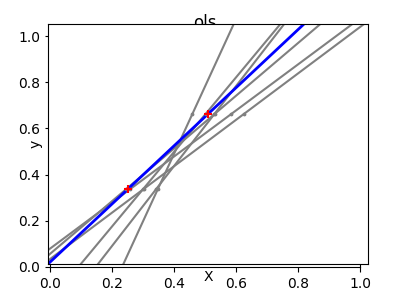>>> X = np.c_[ .5, 1].T
>>> y = [.5, 1]
>>> test = np.c_[ 0, 2].T
>>> regr = linear_model.LinearRegression()

>>> import matplotlib.pyplot as plt
>>> plt.figure()

>>> np.random.seed(0)
>>> for _ in range(6):
...    this_X = .1*np.random.normal(size=(2, 1)) + X
...    regr.fit(this_X, y)
...    plt.plot(test, regr.predict(test))
...    plt.scatter(this_X, y, s=3)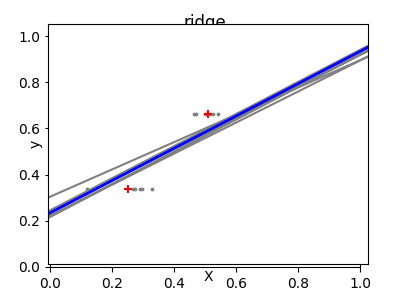>>> regr = linear_model.Ridge(alpha=.1)

>>> plt.figure()

>>> np.random.seed(0)
>>> for _ in range(6):
...    this_X = .1*np.random.normal(size=(2, 1)) + X
...    regr.fit(this_X, y)
...    plt.plot(test, regr.predict(test))
...    plt.scatter(this_X, y, s=3)


>>> alphas = np.logspace(-4, -1, 6)
>>> from __future__ import print_function
>>> print([regr.set_params(alpha=alpha
...             ).fit(diabetes_X_train, diabetes_y_train,
...             ).score(diabetes_X_test, diabetes_y_test) for alpha in alphas])
[0.5851110683883..., 0.5852073015444..., 0.5854677540698..., 0.5855512036503..., 0.5830717085554..., 0.57058999437...]


### 稀疏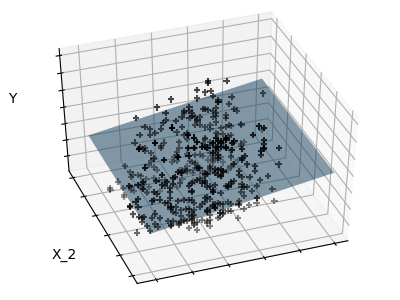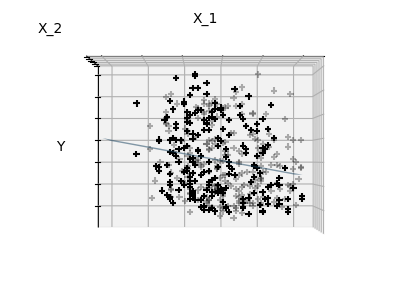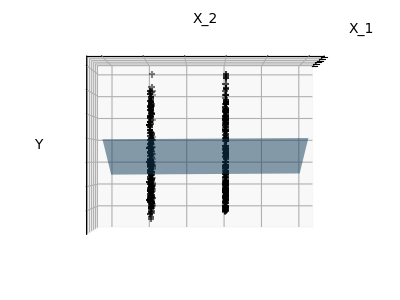>>> regr = linear_model.Lasso()
>>> scores = [regr.set_params(alpha=alpha
...             ).fit(diabetes_X_train, diabetes_y_train
...             ).score(diabetes_X_test, diabetes_y_test)
...        for alpha in alphas]
>>> best_alpha = alphas[scores.index(max(scores))]
>>> regr.alpha = best_alpha
>>> regr.fit(diabetes_X_train, diabetes_y_train)
Lasso(alpha=0.025118864315095794, copy_X=True, fit_intercept=True,
max_iter=1000, normalize=False, positive=False, precompute=False,
random_state=None, selection='cyclic', tol=0.0001, warm_start=False)
>>> print(regr.coef_)
[   0.         -212.43764548  517.19478111  313.77959962 -160.8303982    -0.
-187.19554705   69.38229038  508.66011217   71.84239008]


### 分类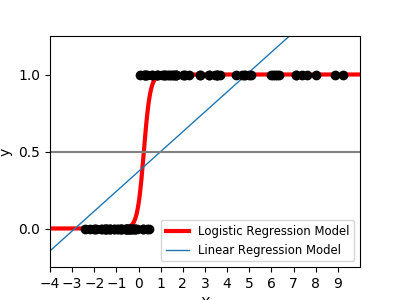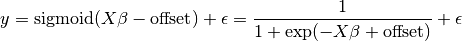>>> logistic = linear_model.LogisticRegression(C=1e5)
>>> logistic.fit(iris_X_train, iris_y_train)
LogisticRegression(C=100000.0, class_weight=None, dual=False,
fit_intercept=True, intercept_scaling=1, max_iter=100,
multi_class='ovr', n_jobs=1, penalty='l2', random_state=None,
solver='liblinear', tol=0.0001, verbose=0, warm_start=False)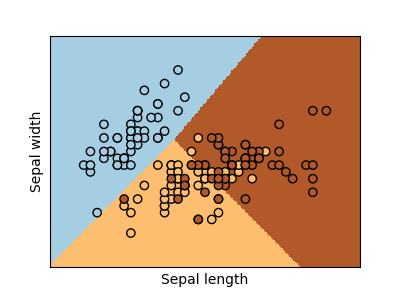LogisticRegression 对象中的 C 参数控制着正则化数量：C 值越大，正则化数量越小。penalty="l2" 提供收缩(比如，无稀疏系数)，同时 penalty=”l1” 提供稀疏化

from sklearn import datasets, neighbors, linear_model
X_digits = digits.data
y_digits = digits.target


## 支持向量机(SVMs)

### 线性 SVMs

SVMs 可以用于回归：SVR (支持向量回归)–，或者分类 SVC (支持向量分类)。

>>> from sklearn import svm
>>> svc = svm.SVC(kernel='linear')
>>> svc.fit(iris_X_train, iris_y_train)
SVC(C=1.0, cache_size=200, class_weight=None, coef0=0.0,
decision_function_shape='ovr', degree=3, gamma='auto', kernel='linear',
max_iter=-1, probability=False, random_state=None, shrinking=True,
tol=0.001, verbose=False)


### 使用核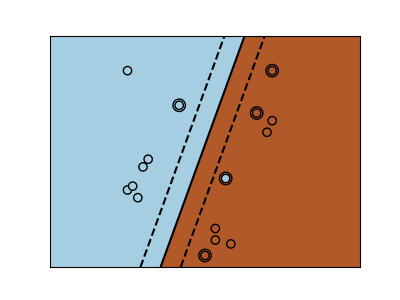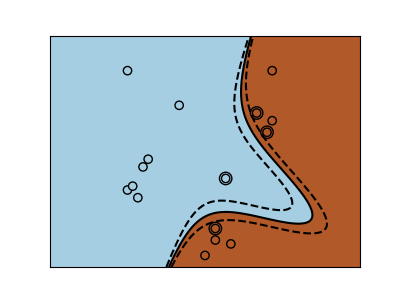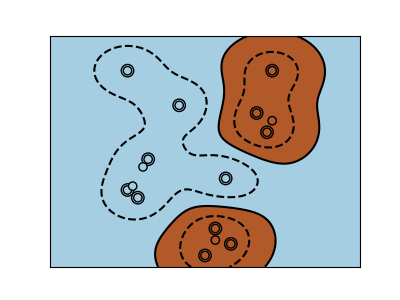>>> svc = svm.SVC(kernel='linear') >>> svc = svm.SVC(kernel='poly',degree=3) >>> svc = svm.SVC(kernel='rbf')

iris = datasets.load_iris()
X = iris.data
y = iris.target
X = X[y != 0, :2]
y = y[y != 0]